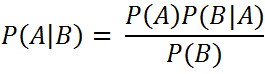`  I request GUEST WRITERS for this web site. Anyone interested please contact me at saravu2k2013@gmail.com Thanks  `
Showing posts with label Hidden Markov Model. Show all posts
Showing posts with label Hidden Markov Model. Show all posts

## Wednesday, 6 November 2019

### Simple introduction to Bayes theorem and Hidden markov model

Simple and effective introduction to Bayes theorem and Hidden Markov Model (HMM)

Luis Serrano has explained the underlying concepts of Bayes theorem and HMM  in a friendly manner in this video lecture.

Bayes theorem: It is a probabilistic approach used to calculate the probability of an event given the probability of another associated event.

Hidden Markov Model (HMM): It is a class of probabilistic models that helps us in unveiling a hidden sequence of events (finite set of events each representing a state) using an observed sequence of events (each observed event is emitted by a hidden state)